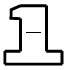### Home > CC1 > Chapter 3 > Lesson 3.1.1 > Problem3-12

3-12.

Copy and complete each of the following Giant One problems.

1.  $\frac{5}{3}\cdot$$=\ \frac{ }{18}$

• By what number do you multiply $3$ to get $18$? This will be both the numerator and denominator in the Giant One, since the fraction is equal to one.

$\left(\frac{5}{3}\right) \! \left(\frac{6}{6}\right) \! =\frac{30}{18}$

1.  $\frac{28}{63}=$$\cdot\ \frac{4}{ }$

Follow the same method as in part (a). What number times $4$ is equal to $28$?

1.  $\frac{9}{20}\cdot$$=\ \frac{ }{100}$

This problem is very similar to part (a). Remember that the Giant One is a fraction equal to one.